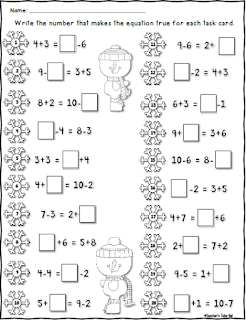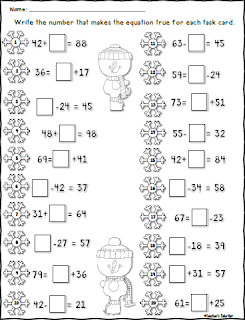# Winter Equal Equations and a Freebie

How many of your students struggle with equal equations? How many of your students are still not sure about that equal sign? I have created 3 different packs to help struggling students; Single Digit Equal Equations, 2-Digit Missing Numbers using Addition and Subtraction, and Equal Equations using Multiplication and Division.

Each pack includes a worksheet and 30 task cards. I am proving the worksheet from each pack right here for FREE! There are 3 different Worksheets to choose from. Choose the worksheet that fits the needs of your classroom.

Equal Equations can be really hard for some students. These task cards and worksheets will help students practice equations with regard to the equal sign. Your math centers will come alive with these winter theme snowmen task cards.

Here is the Multiplication and Division Equal Equations:

Here is the Single Digit Addition and Subtraction Task Cards. This pack uses simple equations for the beginner! Click the Worksheet below to grab this freebie!Here is the 2-Digit Addition and Subtraction Missing Numbers Task Cards and Worksheet. Students need to pay attention to the equal sign in order to find the correct missing number. Click the worksheet to download this freebie!Here's to equal equations!
Happy Holidays,